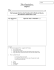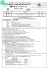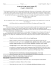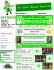Assignment 2 the 1.

Transcription

Assignment 2 the 1.
Assignment 2
(Be sure to observe the rules about handing in homework)
x1  x 2  x3  3
1.
Solve:
6 x1  2 x 2  2 x3  2
 3x1  4 x 2  x3  1
with
(a) (10 pts) naive Gauss elimination (no pivoting),
(b) (10 pts) Gauss with partial pivoting
*You need to show all of the steps manually. MATLAB code alone is not acceptable.
2. (a) (10 pts) Solve the following system of equations by LU decomposition without pivoting.
Show explicitly the L and U matrices found during the process.
8 x1  4 x 2  x3  11
 2 x1  3x 2  x3  4
2 x1  x 2  6 x3  7
(b) (15 pts) Determine the matrix inverse in three steps (column by column) as taught in class.
Check your results by verifying that [A][A]-1 = [I]. You may use MATLAB to verify your results.
3. Use least-squares regression to fit a straight line to
x
y
1
1
2
1.5
3
2
4
3
5
4
6
5
7
8
8
10
9
13
(a) (7 pts) Along with the slope and intercept, compute Sr, St, and the correlation coefficient r as
described in lecture slides (slide#9 in Chap-17e.ppt).
Plot the data and the straight line. Assess the fit.
(b) (8 pts) Recompute (a), but use polynomial regression to fit a parabola to the data. Compare
the results with those of (a) using the correlation coefficient r.
Which one (a line or a parabola?) does provide a better fit?
4. Given the data
x
y
5
17
10
24
15
31
20
33
25
37
30
37
35
40
40
40
45
42
50
41
use least-squares regression to fit
(a) (10 pts) a power equation, and
(b) (10 pts) a saturation-growth-rate equation.
Plot the data along with all the curves. Is any one of the curves superior? If so, justify by using the
correlation coefficient r.
(* Note that correlation coefficient r must be calculated using the original data and the
fitted non-linear function. In other words, do not use the transformed values for the
calculation of the correlation coefficient *)
(*Important Note: Do not use package programs to find the answers. However, you may use the
5. (10 pts) Given the data
x
f (x)
1
3
2
6
3
19
5
99
7
291
8
444
Calculate f(4) using Newton’s interpolating polynomials of order 1 through 4, i.e. you will
calculate f1(4), f2(4), f3(4), and f4(4). In each case, choose your base points to attain good accuracy
(Hint: around the point of interest, i.e. 4). What do your results indicate regarding the order of the
polynomial used to generate the data in the table?
6. (10 pts) Repeat the question above (Q.5) using Lagrange polynomials of order 1 through 3.

Problem Set 3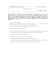Exam 3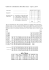10.1\\\-10.3 review ans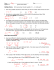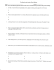Automata Theory Sample Midterm Exam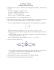Math 0120 Examination #3 Sample Name (Print)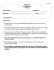Math 30G – Calculus – Sample Final Exam Fall 2012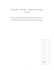Blank PDF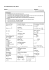3w 3 t5+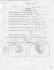CPSC 636-600 Homework 4 (Total of 100 points)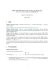Sample Exam 3 - People Pages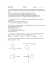2 for 1 Dinner Menu - Club Regent Casino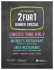Name _____Mr. Perfect________________________________ Date __F 14_______ n l of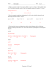Cover Sheet! EXAM2 PRINT your name legibly on the line below.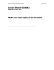Questions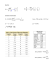Exam 3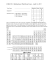Elementary Algebra Sample Test #1 1. 2.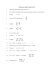AP Chemistry Summer Work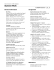The Outsiders: - Mr. Sturgeon`s Wonderful World of English